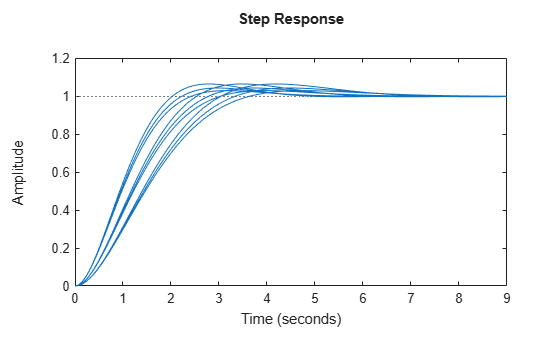# Model Array with Variations in Two Parameters

This example shows how to create a two-dimensional (2-D) array of transfer functions using `for` loops. One parameter of the transfer function varies in each dimension of the array.

You can use the technique of this example to create higher-dimensional arrays with variations of more parameters. Such arrays are useful for studying the effects of multiple-parameter variations on system response.

The second-order single-input, single-output (SISO) transfer function

`$H\left(s\right)=\frac{{\omega }^{2}}{{s}^{2}+2\zeta \omega s+{\omega }^{2}}.$`

depends on two parameters: the damping ratio, $\zeta$, and the natural frequency, $\omega$. If both $\zeta$ and $\omega$ vary, you obtain multiple transfer functions of the form:

`${H}_{ij}\left(s\right)=\frac{{\omega }_{j}^{2}}{{s}^{2}+2{\zeta }_{i}{\omega }_{j}s+{\omega }_{j}^{2}},$`

where ${\zeta }_{i}$ and ${\omega }_{j}$ represent different measurements or sampled values of the variable parameters. You can collect all of these transfer functions in a single variable to create a two-dimensional model array.

Preallocate memory for the model array. Preallocating memory is an optional step that can enhance computation efficiency. To preallocate, create a model array of the required size and initialize its entries to zero.

`H = tf(zeros(1,1,3,3));`

In this example, there are three values for each parameter in the transfer function H. Therefore, this command creates a 3-by-3 array of single-input, single-output (SISO) zero transfer functions.

Create arrays containing the parameter values.

```zeta = [0.66,0.71,0.75]; w = [1.0,1.2,1.5];```

Build the array by looping through all combinations of parameter values.

```for i = 1:length(zeta) for j = 1:length(w) H(:,:,i,j) = tf(w(j)^2,[1 2*zeta(i)*w(j) w(j)^2]); end end```

`H` is a 3-by-3 array of transfer functions. $\zeta$ varies as you move from model to model along a single column of `H`. The parameter $\omega$ varies as you move along a single row.

Plot the step response of `H` to see how the parameter variation affects the step response.

`stepplot(H)`You can set the `SamplingGrid` property of the model array to help keep track of which set of parameter values corresponds to which entry in the array. To do so, create a grid of parameter values that matches the dimensions of the array. Then, assign these values to `H.SamplingGrid` with the parameter names.

```[zetagrid,wgrid] = ndgrid(zeta,w); H.SamplingGrid = struct('zeta',zetagrid,'w',wgrid);```

When you display `H`, the parameter values in `H.SamplingGrid` are displayed along with the each transfer function in the array.